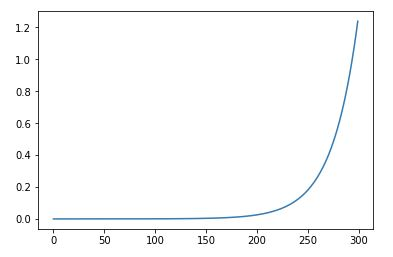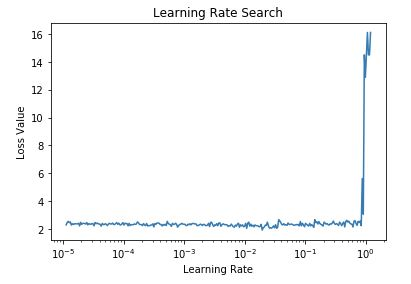# Wiki: Lesson 2

How did you place your own data to test? I cannot figure it out.

Make sure you include the test data in ImageClassifier data by passing test_name = ‘name of the test folder name’ then predict the test images by calling the predict function with argument is_test = ‘True’

``````data = ImageClassifierData.from_paths(PATH,test_name ='test',tfms=tfms_from_model(arch,sz));
Preds = np.exp(learn.predict(is_test = True)``````

At 1:42:46 Jeremy reduces the steps to create the model and in those steps, he also instructs to train the frozen network first then train the unfrozen network.

I do not understand what he means by training a frozen and unfrozen network. In general, I am pretty confused about freezing and un-freezing networks. Please help.

The first thing to understand here is that we are using transfer learning. So, we take weights of resnext50(trained on imagenet), which is frozen by default, and replace the last layer with a custom one (which is done for you by the fastai library, and also, its very important to understand why are we doing this and how does this look like for our model…).

What jeremy means by frozen is, we are not gonna train the downloaded weights (no gradient update takes place during back-prop), for the frozen layers. So, here we are training only the custom layer that we have attached. You can see that it takes very less time to train in this case, as we are learning* only the last layer.

By unfreezing these layers and training’em, we are updating all the weights in our network ( pretrained resnext weights + custom head weights ). So, when you do learn.unfreeze(), you can notice the increase in gpu consumption.

Hope, this is clear.

7 Likes

Thank you so much. It’s much clear now.

1 Like

## Check your understanding of the lesson 2

(post original in portuguese at Deep Learning Brasilia - Lição 2)

Hi guys,

I did watch again the video of the lesson 2 (part 1) to get the whole image and I took notes of the vocabulary used by @jeremy.

Let’s play ! OK ?Can you give a definition / a url / an explanation for all the followings terms and expressions ?

If yes, you are done with the 2nd lesson !!!PS : you do not want to test yourself or you want to check your answers ? Go to the blog post “Deep Learning 2: Part 1 Lesson 2” of @hiromi : " super travail !!!"

• Image classifier
• 3 lines of code
• the key point to train the model is the PATH to data
• particular structure of the data folder with train, validation, test folders
• each folder with subfolders cats and dogs
• pretty standard data folder stucture
• validation accuracy
• image vizualisation
• learning rate
• epoch
• dataset
• training set loss
• validation set loss
• Deep Learning
• minimum point of a function
• function which has hundreds of million parameters
• algorithm
• what is the loss or error at this point choosen at random
• what is the gradient at this point
• learning rate
• learning rate finder
• mini batch
• GPU
• parallel processing power of the GPU effectively (generally 64 or 128 images at a time)
• mini batch = iteration
• learn.lr_find()
• learn.sched.plot()
• 10-1 = 1e-1
• we are not looking at the point with the lowest learning rate
• hyper parameters
• fastai library
• momentum
• learning rate optimizer
• dynamic learning rate
• make your model better : give more data
• overfitting
• specialization of the model
• the model hast to generalize
• more labeled data
• data augmentation
• rotation, flip, zoom
• aug_tfms
• tfms_from_model
• transforms_side_on
• transforms_top_down
• each type of image has a particular data ugmentation
• learning rate versus loss
• the higher learning rate that gives the lower loss
• all local mimina are the same
• unfreezing layers
• precompute=True
• activation is a number (feature, level of confidence, probability)
• save the activations for all images of the dataset
• first time you train the model, it is longer than after when precompute=True
• when precompute=true, data augmentation does not have an impact
• learn.precompue=False
• overfitting = your model does not generalize
• stochastisc gradient descent with restarts
• cycle_len=1
• learning rate annealing
• cosine annealing
• learning rate schedule
• learn.sched.plot_lr()
• with SGDR, you do not need to retrain your model with new random values of the model parameters
• even better for generalization, you can save parameters values befor the restart and get the average of them (cycle_save_name)
• learn.save()
• each time you create a new object learn, you start with a new model with new sets of weights
• weights / parameters
• fine tuning
• pretrained model
• we’ve learned new layers on top of the pretrained model
• frozen layers are layers not trained
• learn.unfreeze()
• layer 1 looks for edges and gradients
• the early layers need no training or a little
• diferential learning rates
• lr = array([lr1,lr2,lr3])
• if images look like the imagenet ones, divide by 10. If not, divide by 3.
• learn.fit(lr, 3, cycle_len=1, cycle_mult=2)
• size of images
• learn.freeze_to (if you want to freeze a particular layer)
• number of cycles
• cycle_mult multiplies the length of a cycle after each training through a cycle
• hyper parameters
• all our inout images mut be squared in terms of dimensions as the dimensions have to be consistent to be computed by the GPU
• predictions on validation set
• TTA : Test Time Augmentation on validation or test data set when you want to get the prediction values (take the average of predictions after data augmentation)
• log_preds,y=learn.TTA()
• accuracy(log_preds,y)
• cropping images is not recommended
• fastai library is Open Source
• pytorch
• library on top of pytorch because pytorch is not so easy to use
• model to phone, tensorflow
• confusion matrix
• plot_confusion_matrix(cm, data.classes)
• training a world class image classifier in 8 steps (there are 3 main steps in fact)
• dogbreed kaggle competition
• kaggle cli script
• pandas
• csv files
• a pivot table
• max_zoom : random zoom up to the choosen number (like 1.1)
• cross validation indexes
• val_idxs = get_cv_idxs(n)
• mostly imagenet images size is 224 x 224 or 299 x 299
• dictionary comprehension
• list comprenhension
• matplotlib
• histogram
• size of validation set
• when I start training a model, I want to do it very fast : so, I need small images (like 224 x 224 or smaller)
• CUDA memory error (you should decrease your batch size)
• Kernel restart
• the more classes you have and the more difficult to get an accuracy very high
• if you train something on a smaller size, you can call learn.set_data(get_data(299,bz)) and pass in a larger dataset
• learn.freeze()
• fully convolutional architectures can handle pretty much arbitrary sizes
• underfitting means that cycle_len is too short
• dataset balanced or unbalanced
• mainly 3 steps : search for the learning rate, train with frozen layers, train with unfrozen layers
• improve the training by increasing image sizes and get a better architecture
• deconvolution
• resnet34
• resnext50
• satelite images (Planet dataset)
• learn.sched.plot_loss()
• Crestle
• Paperspace
• AWS, AWS credit
• AWS setup : console, EC2, launch an instance, Community AMIs, fastai, p2.xlarge, launch, key pair, Public Ip adress, ssh
• cd fastai
• git pull
• conda env update
• don’t forget stopping your GPU on Crestle/Paperspace/AWS !
3 Likes

That’s great @pierreguillou - thanks!1 Like

little confusion here, if precompute=False while using data augmentation, does activations for new augmented picture of say cat are computed or not?? or same activations for augmented image is used as of orignal image or agumentation is ignored??

That helps a lot. Can you explain how precompute works? Why train the model with `precompute=True` when you’re just going to set `precompute=False` before training with transformations? I know Jeremy talks about this in the video, but I don’t understand why `precompute=True` does not take into account the transformations.

EDIT : In this post I’ve described my intuition on what `precompute=true` does. Sorry, for this erroneous explanation, as it is quite misleading.Thank you @jeremy , for pointing it out. Please, check out this post Wiki: Lesson 2 , for more clarity. Also, precompute in fastai .

Pre-compute is a kind of a hack, Jeremy engineered to incorporate augmentation.
Image augmentation artificially creates training images through different ways of processing or combination of multiple processing, such as random rotation, flips, shifts e.t.c. Suppose we create five different augmentations for each image and if we have 10 GB of training images. We will end up with 50GB+ data. So, precompute=false means that we don’t generate these augmented examples. Computation-wise, generating these (in ram) is fast but storing it in hardisk is a slower process. Now, storing time (ram to hardisk) for these 50gb+ data is very high comparitively. When precompute=true, these augmented images are generated during training and sent to model(in ram/vram), then it’s deleted. This is an optimized way of computation.
This is my understanding.
Understanding concepts behind iterable, iterator and generator will help in implementing optimizations, like these.

I’m having the same issue. How do I set up this data?

Hello ,
I am trying to code the Dog Breed notebook. I am getting an error when I try to execute
learn = ConvLearner.pretrained(arch,data,precompute=True).
The last line of error trace shows [Errno 2] No such file or directory : ‘/home/paperspace/fastai/courses/dl1/fastai/weights/resnext101_64x4d.pth’

Hi Bikash

this post should help:

@mhmoodlan
Cyclical Learning Rates for Training Neural Networks
Link to to paper used in learning rate finder: https://arxiv.org/abs/1506.01186

1 Like

Hi,

I’m a little confused on the learning rate finder. Should it be used on an untrained model? I’m trying to implement the algorithm myself in Keras (on an untrained example CNN model – see gist at bottom)

I thought I would write my own learning rate scheduler to feed into a keras model, but when plotting my loss vs learning rate, the loss just hovers around 2 then explodes when the Learning rate gets close to 1.

I start with a learning rate of 1e-5. At the end of each batch, I update my learning rate:

newLR = initialLR* (1 + 0.04)^{batchNumber}

which gives me a similar learning rate scheduling as the lecture notes:When I compare this learning rate to the loss values at the end of each batch, I get a relatively flat loss value then it explodes:Not sure what I’m missing here… I created a Gist of the code I used to code this up. Most of the action happens in the `def on_batch_end` function in my callback.

I expected to see the loss decrease in a nice downhill kind of way to a valley, the way the learning rate vs loss is shown in the lecture, but yeah mine isn’t even close to that.

Any advice would be much appreciated!

when run lesson1-rxt50.ipynb I get ‘invalid index to scalar variable.’ in function save_metrics after learn.fit()
can somebody help me,thanks

I have the same issue. There is an double “” in sgdr.py:79
If you change it to single, it will work correctly.

But anyway I think there should be a conditional loop through vals, to support multiple or single val losses,
.fit(…, all_val=True) will pass here multi val losses.

1 Like

This isn’t what happens. No data augmentation occurs when `precompute=True`. Search the forums for “precompute” to see the correct answer to this question.

1 Like

I try to use .fit(…, all_val=True),another issue came up.
I change the source code a lit by add some if/else to avoid the scalar vals to be indexed.
thank you very much for you answer.